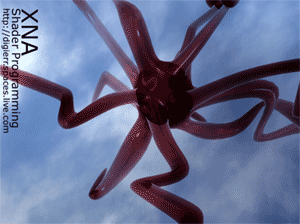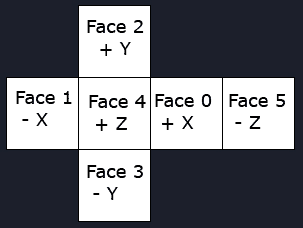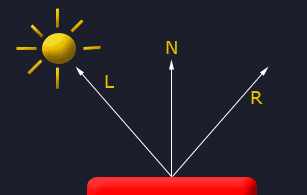## 动态环境映射R = 2 * N·L * N - Ltexture ReflectionCubeMap;
samplerCUBE ReflectionCubeMapSampler = sampler_state
{
texture = <ReflectionCubeMap>;
};


float Diff = saturate(dot(L, N));

// Calculate reflection vector
float3 Reflect = normalize(2 * Diff * N - L);  

float3 ReflectColor = texCUBE(ReflectionCubeMapSampler, Reflect);

return Color*vAmbient*float4(ReflectColor,1) + Color*vDiffuseColor *
Diff*float4(ReflectColor,1) + vSpecularColor * Specular*float4(ReflectColor,1);	

RenderTargetCube RefCubeMap;
TextureCube EnvironmentMap;  

RefCubeMap = new RenderTargetCube(this.GraphicsDevice, 256, 1, SurfaceFormat.Color);

RenderTargetCube函数需要graphics device对象，每个纹理的大小(这里是256x256 )，mipmap的级别和SurfaceFormat。

effect.Parameters["ReflectionCubeMap"].SetValue(EnvironmentMap);

for (int i = 0; i < 6; i++)
{
// render the scene to all cubemap faces
CubeMapFace cubeMapFace = (CubeMapFace)i;
switch (cubeMapFace)
{
case CubeMapFace.NegativeX:
{
viewMatrix = Matrix.CreateLookAt(Vector3.Zero, Vector3.Left, Vector3.Up);
break;
}
case CubeMapFace.NegativeY:
{
viewMatrix = Matrix.CreateLookAt(Vector3.Zero, Vector3.Down, Vector3.Forward);
break;
}
case CubeMapFace.NegativeZ:
{
viewMatrix = Matrix.CreateLookAt(Vector3.Zero, Vector3.Backward, Vector3.Up);
break;
}
case CubeMapFace.PositiveX:
{
viewMatrix = Matrix.CreateLookAt(Vector3.Zero, Vector3.Right, Vector3.Up);
break;
}
case CubeMapFace.PositiveY:
{
viewMatrix = Matrix.CreateLookAt(Vector3.Zero, Vector3.Up, Vector3.Backward);
break;
}
case CubeMapFace.PositiveZ:
{
viewMatrix = Matrix.CreateLookAt(Vector3.Zero, Vector3.Forward, Vector3.Up);
break;
}
}
effect.Parameters["matWorldViewProj"].SetValue(worldMatrix * viewMatrix * projMatrix);
// Set the cubemap render target, using the selected face
this.GraphicsDevice.SetRenderTarget(0, RefCubeMap, cubeMapFace);
this.GraphicsDevice.Clear(Color.White); this.DrawScene(false);
}
graphics.GraphicsDevice.SetRenderTarget(0, null);
this.EnvironmentMap = RefCubeMap.GetTexture();


2006 - 2023，推荐分辨率1024*768以上，推荐浏览器Chrome、Edge等现代浏览器，截止2021年12月5日的访问次数：1872万9823 站长邮箱